Скачать презентацию Fi 8000 Valuation of Financial Assets Fall Semester

3163c7e7d6bb3b137fbe1d9d23d0cc72.ppt

• Количество слайдов: 37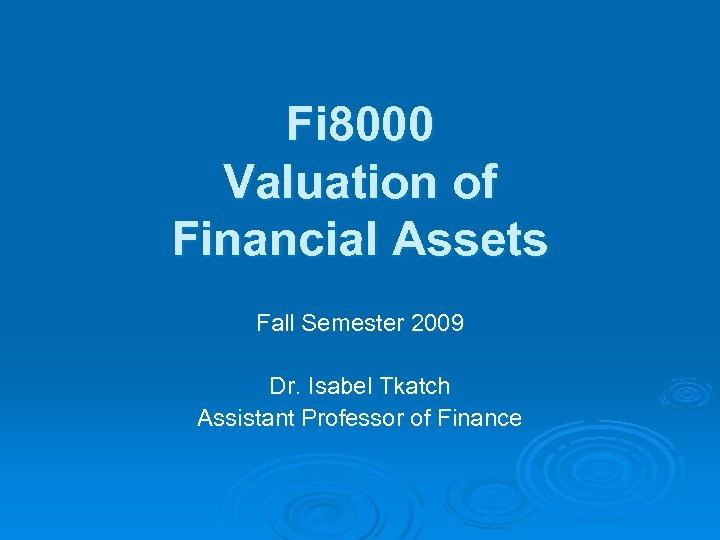Fi 8000 Valuation of Financial Assets Fall Semester 2009 Dr. Isabel Tkatch Assistant Professor of Finance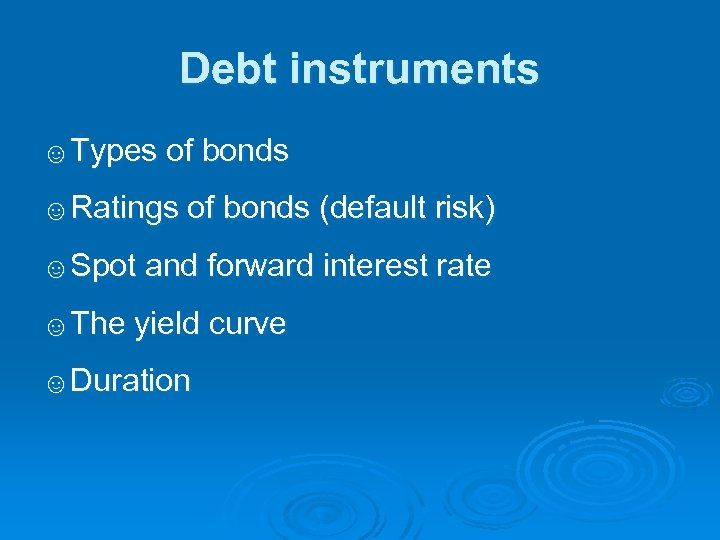Debt instruments ☺Types of bonds ☺Ratings of bonds (default risk) ☺Spot and forward interest rate ☺The yield curve ☺Duration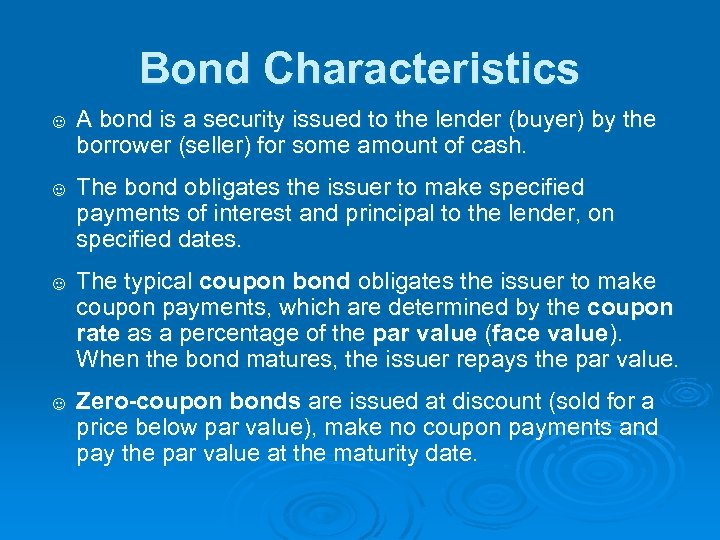Bond Characteristics ☺ A bond is a security issued to the lender (buyer) by the borrower (seller) for some amount of cash. ☺ The bond obligates the issuer to make specified payments of interest and principal to the lender, on specified dates. ☺ The typical coupon bond obligates the issuer to make coupon payments, which are determined by the coupon rate as a percentage of the par value (face value). When the bond matures, the issuer repays the par value. ☺ Zero-coupon bonds are issued at discount (sold for a price below par value), make no coupon payments and pay the par value at the maturity date.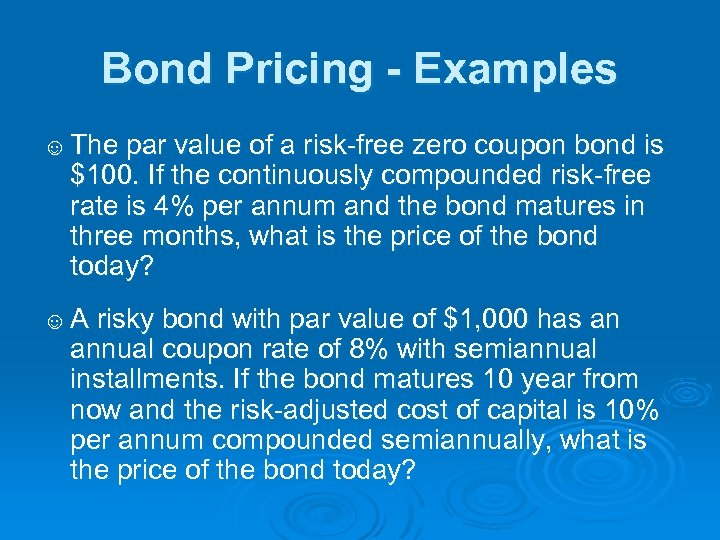Bond Pricing - Examples ☺ The par value of a risk-free zero coupon bond is \$100. If the continuously compounded risk-free rate is 4% per annum and the bond matures in three months, what is the price of the bond today? ☺ A risky bond with par value of \$1, 000 has an annual coupon rate of 8% with semiannual installments. If the bond matures 10 year from now and the risk-adjusted cost of capital is 10% per annum compounded semiannually, what is the price of the bond today?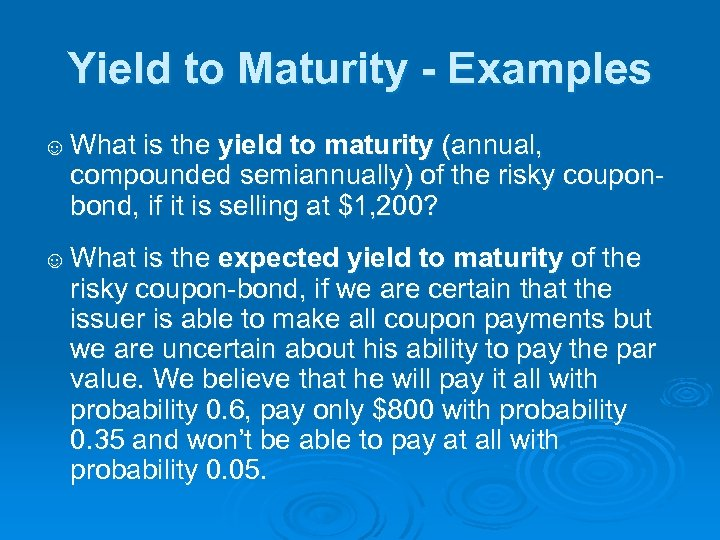Yield to Maturity - Examples ☺ What is the yield to maturity (annual, compounded semiannually) of the risky couponbond, if it is selling at \$1, 200? ☺ What is the expected yield to maturity of the risky coupon-bond, if we are certain that the issuer is able to make all coupon payments but we are uncertain about his ability to pay the par value. We believe that he will pay it all with probability 0. 6, pay only \$800 with probability 0. 35 and won’t be able to pay at all with probability 0. 05.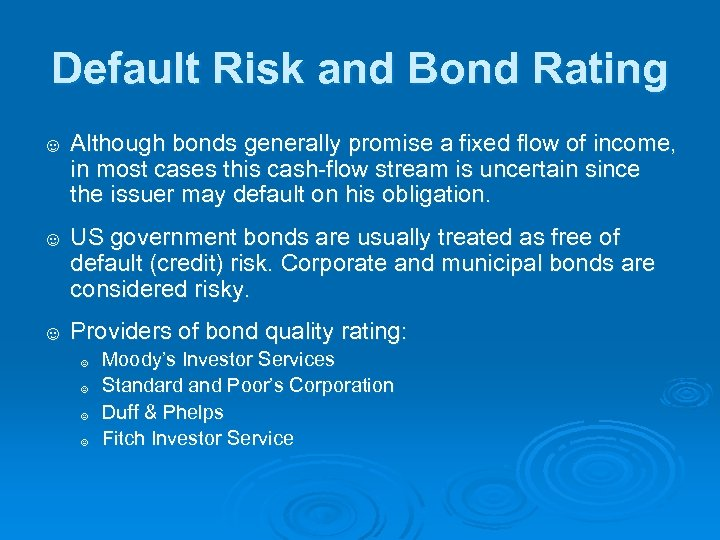Default Risk and Bond Rating ☺ Although bonds generally promise a fixed flow of income, in most cases this cash-flow stream is uncertain since the issuer may default on his obligation. ☺ US government bonds are usually treated as free of default (credit) risk. Corporate and municipal bonds are considered risky. ☺ Providers of bond quality rating: ☺ ☺ Moody’s Investor Services Standard and Poor’s Corporation Duff & Phelps Fitch Investor Service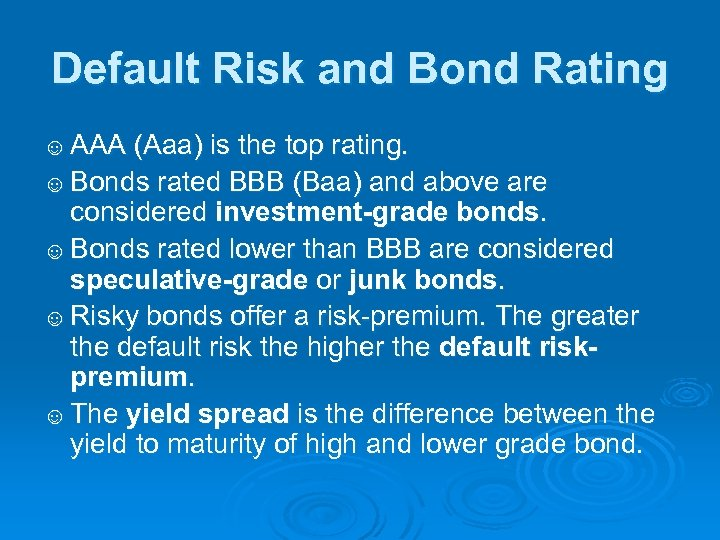Default Risk and Bond Rating ☺ AAA (Aaa) is the top rating. ☺ Bonds rated BBB (Baa) and above are considered investment-grade bonds. ☺ Bonds rated lower than BBB are considered speculative-grade or junk bonds. ☺ Risky bonds offer a risk-premium. The greater the default risk the higher the default riskpremium. ☺ The yield spread is the difference between the yield to maturity of high and lower grade bond.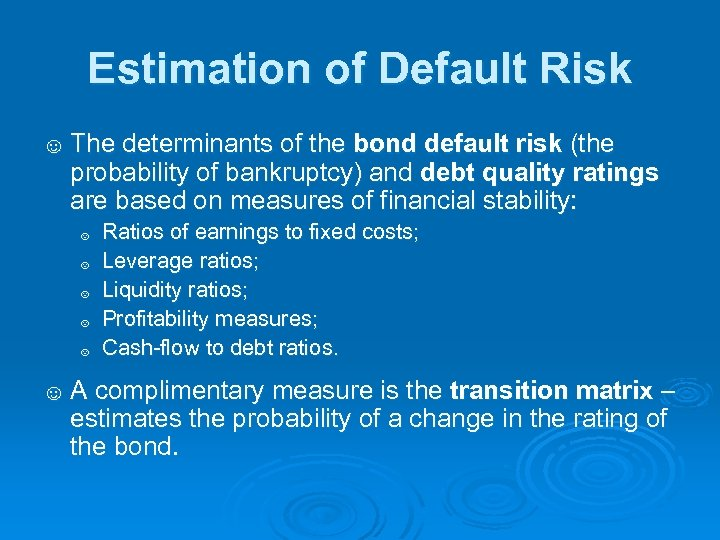Estimation of Default Risk ☺ The determinants of the bond default risk (the probability of bankruptcy) and debt quality ratings are based on measures of financial stability: ☺ ☺ ☺ Ratios of earnings to fixed costs; Leverage ratios; Liquidity ratios; Profitability measures; Cash-flow to debt ratios. ☺ A complimentary measure is the transition matrix – estimates the probability of a change in the rating of the bond.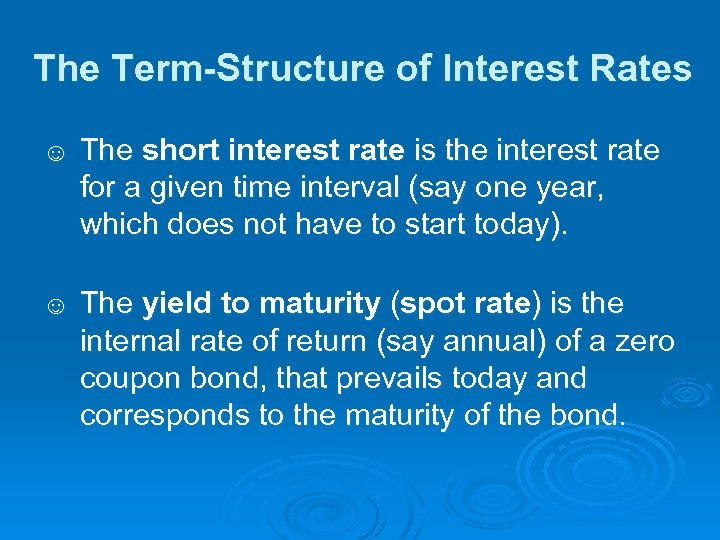The Term-Structure of Interest Rates ☺ The short interest rate is the interest rate for a given time interval (say one year, which does not have to start today). ☺ The yield to maturity (spot rate) is the internal rate of return (say annual) of a zero coupon bond, that prevails today and corresponds to the maturity of the bond.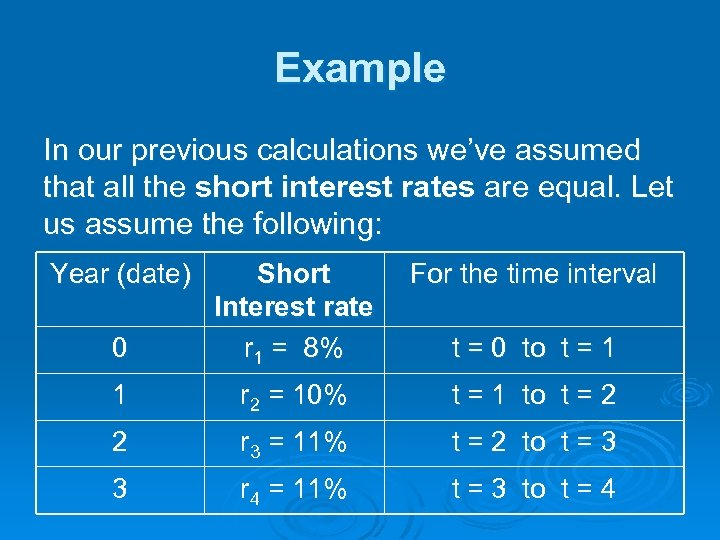Example In our previous calculations we’ve assumed that all the short interest rates are equal. Let us assume the following: Year (date) For the time interval 0 Short Interest rate r 1 = 8% 1 r 2 = 10% t = 1 to t = 2 2 r 3 = 11% t = 2 to t = 3 3 r 4 = 11% t = 3 to t = 4 t = 0 to t = 1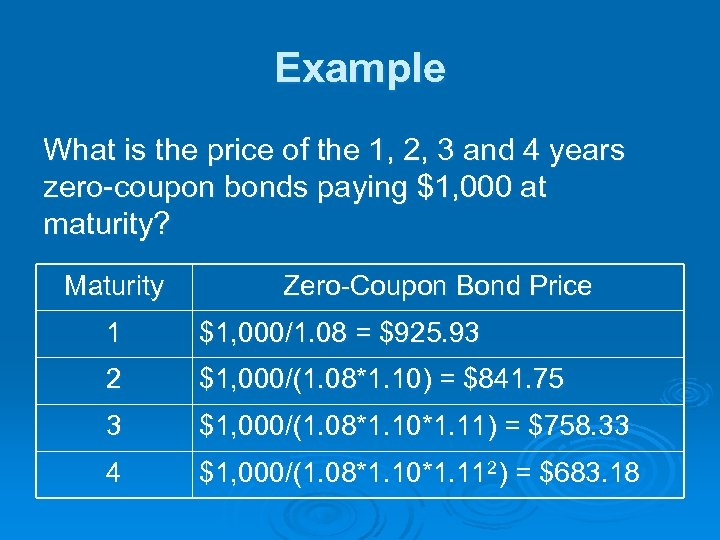Example What is the price of the 1, 2, 3 and 4 years zero-coupon bonds paying \$1, 000 at maturity? Maturity Zero-Coupon Bond Price 1 \$1, 000/1. 08 = \$925. 93 2 \$1, 000/(1. 08*1. 10) = \$841. 75 3 \$1, 000/(1. 08*1. 10*1. 11) = \$758. 33 4 \$1, 000/(1. 08*1. 10*1. 112) = \$683. 18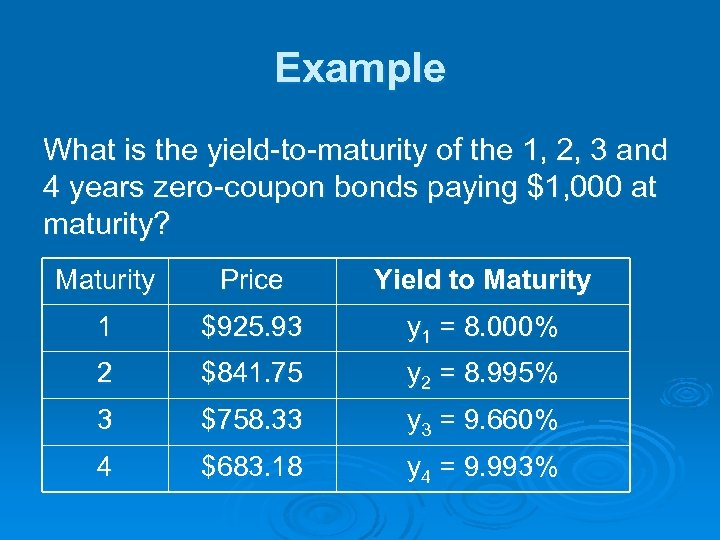Example What is the yield-to-maturity of the 1, 2, 3 and 4 years zero-coupon bonds paying \$1, 000 at maturity? Maturity Price Yield to Maturity 1 \$925. 93 y 1 = 8. 000% 2 \$841. 75 y 2 = 8. 995% 3 \$758. 33 y 3 = 9. 660% 4 \$683. 18 y 4 = 9. 993%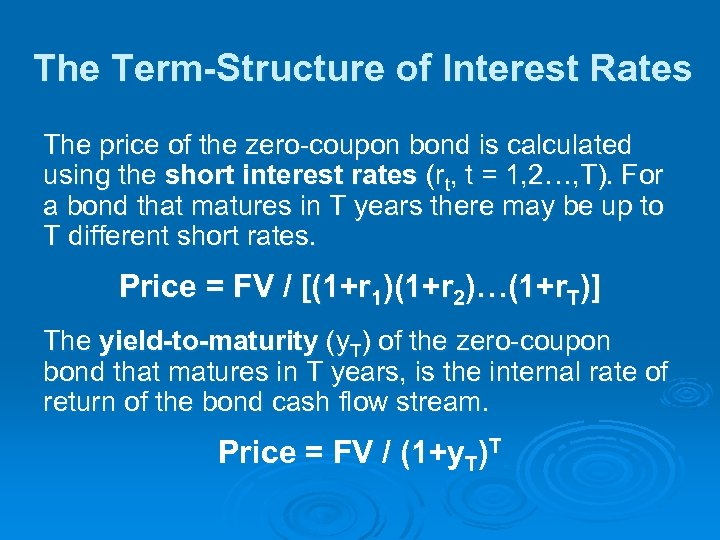The Term-Structure of Interest Rates The price of the zero-coupon bond is calculated using the short interest rates (rt, t = 1, 2…, T). For a bond that matures in T years there may be up to T different short rates. Price = FV / [(1+r 1)(1+r 2)…(1+r. T)] The yield-to-maturity (y. T) of the zero-coupon bond that matures in T years, is the internal rate of return of the bond cash flow stream. Price = FV / (1+y. T)T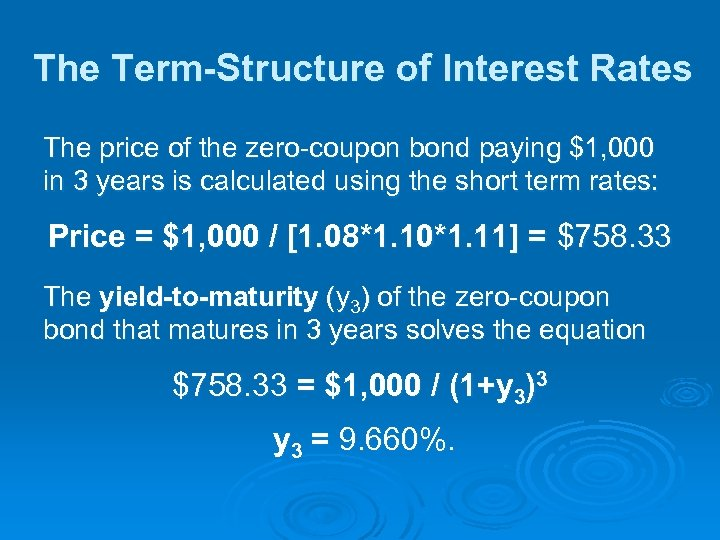The Term-Structure of Interest Rates The price of the zero-coupon bond paying \$1, 000 in 3 years is calculated using the short term rates: Price = \$1, 000 / [1. 08*1. 10*1. 11] = \$758. 33 The yield-to-maturity (y 3) of the zero-coupon bond that matures in 3 years solves the equation \$758. 33 = \$1, 000 / (1+y 3)3 y 3 = 9. 660%.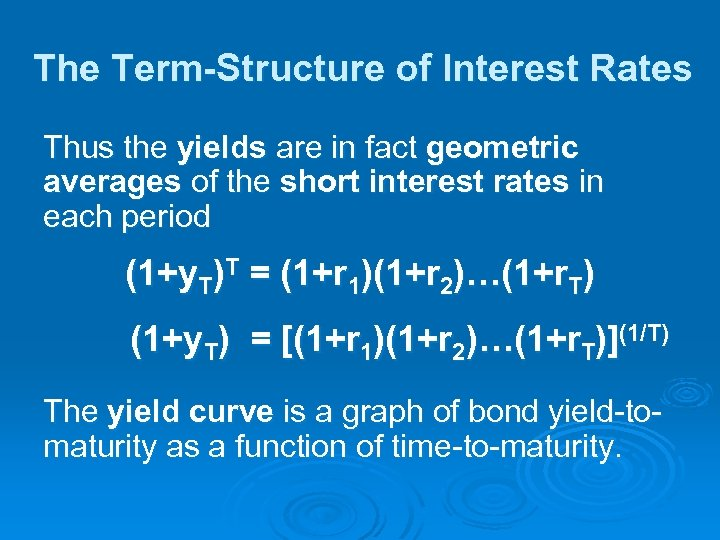The Term-Structure of Interest Rates Thus the yields are in fact geometric averages of the short interest rates in each period (1+y. T)T = (1+r 1)(1+r 2)…(1+r. T) (1+y. T) = [(1+r 1)(1+r 2)…(1+r. T)](1/T) The yield curve is a graph of bond yield-tomaturity as a function of time-to-maturity.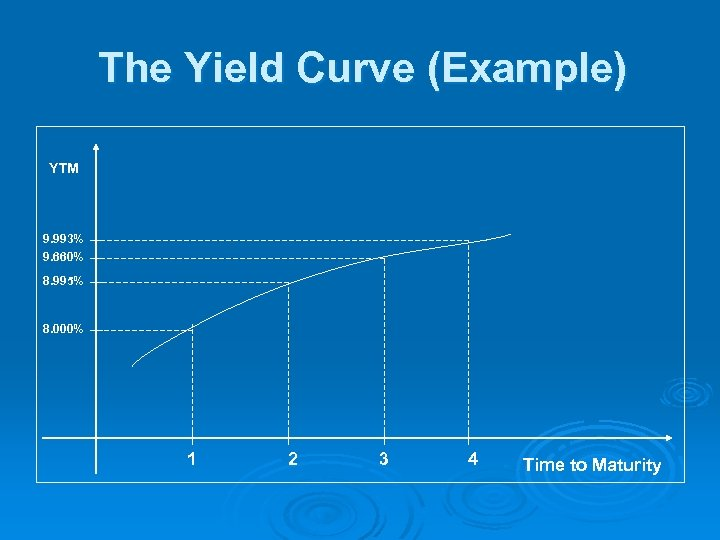The Yield Curve (Example) YTM 9. 993% 9. 660% 8. 995% 8. 000% 1 2 3 4 Time to Maturity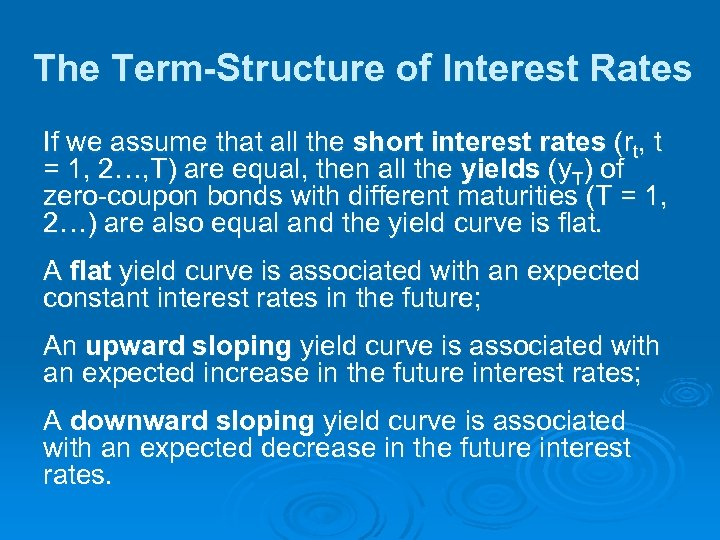The Term-Structure of Interest Rates If we assume that all the short interest rates (rt, t = 1, 2…, T) are equal, then all the yields (y. T) of zero-coupon bonds with different maturities (T = 1, 2…) are also equal and the yield curve is flat. A flat yield curve is associated with an expected constant interest rates in the future; An upward sloping yield curve is associated with an expected increase in the future interest rates; A downward sloping yield curve is associated with an expected decrease in the future interest rates.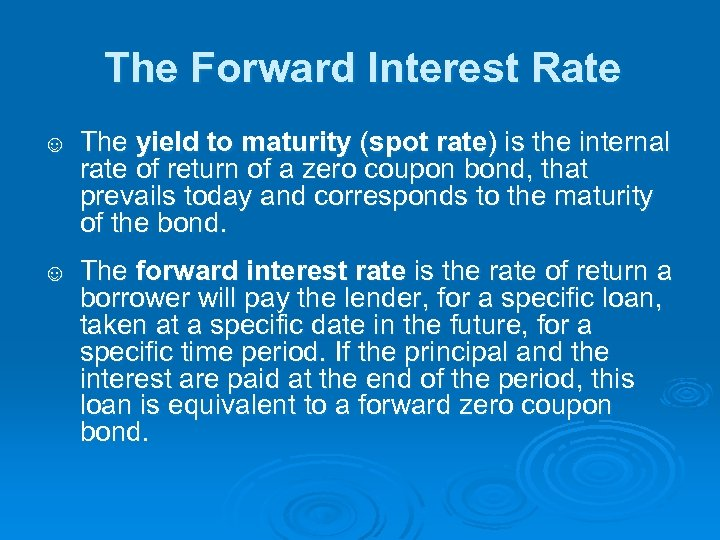The Forward Interest Rate ☺ The yield to maturity (spot rate) is the internal rate of return of a zero coupon bond, that prevails today and corresponds to the maturity of the bond. ☺ The forward interest rate is the rate of return a borrower will pay the lender, for a specific loan, taken at a specific date in the future, for a specific time period. If the principal and the interest are paid at the end of the period, this loan is equivalent to a forward zero coupon bond.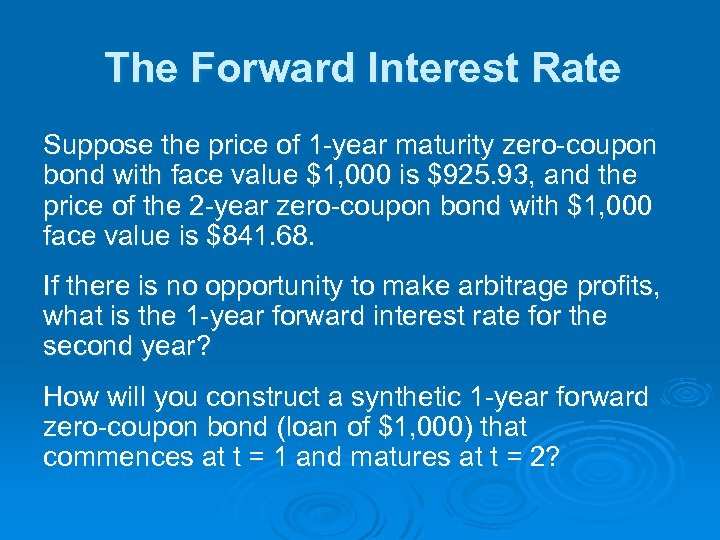The Forward Interest Rate Suppose the price of 1 -year maturity zero-coupon bond with face value \$1, 000 is \$925. 93, and the price of the 2 -year zero-coupon bond with \$1, 000 face value is \$841. 68. If there is no opportunity to make arbitrage profits, what is the 1 -year forward interest rate for the second year? How will you construct a synthetic 1 -year forward zero-coupon bond (loan of \$1, 000) that commences at t = 1 and matures at t = 2?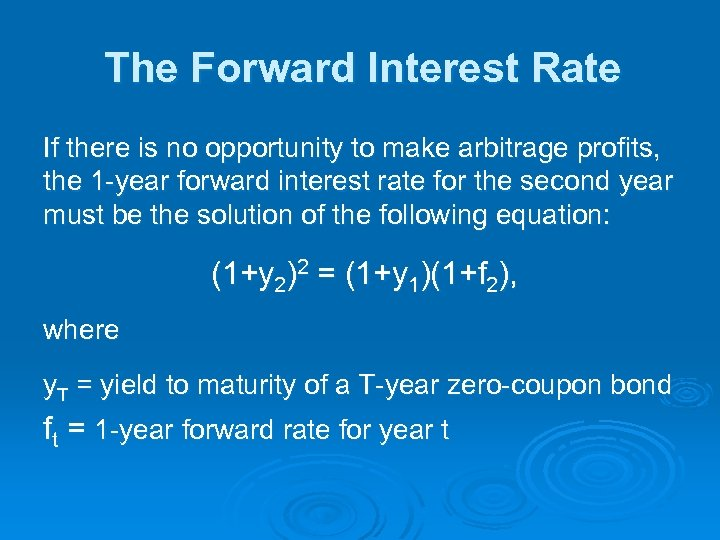The Forward Interest Rate If there is no opportunity to make arbitrage profits, the 1 -year forward interest rate for the second year must be the solution of the following equation: (1+y 2)2 = (1+y 1)(1+f 2), where y. T = yield to maturity of a T-year zero-coupon bond ft = 1 -year forward rate for year t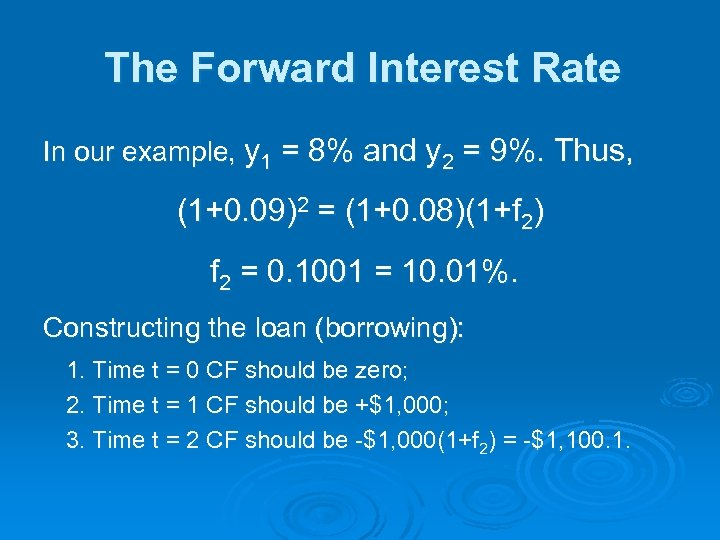The Forward Interest Rate In our example, y 1 = 8% and y 2 = 9%. Thus, (1+0. 09)2 = (1+0. 08)(1+f 2) f 2 = 0. 1001 = 10. 01%. Constructing the loan (borrowing): 1. Time t = 0 CF should be zero; 2. Time t = 1 CF should be +\$1, 000; 3. Time t = 2 CF should be -\$1, 000(1+f 2) = -\$1, 100. 1.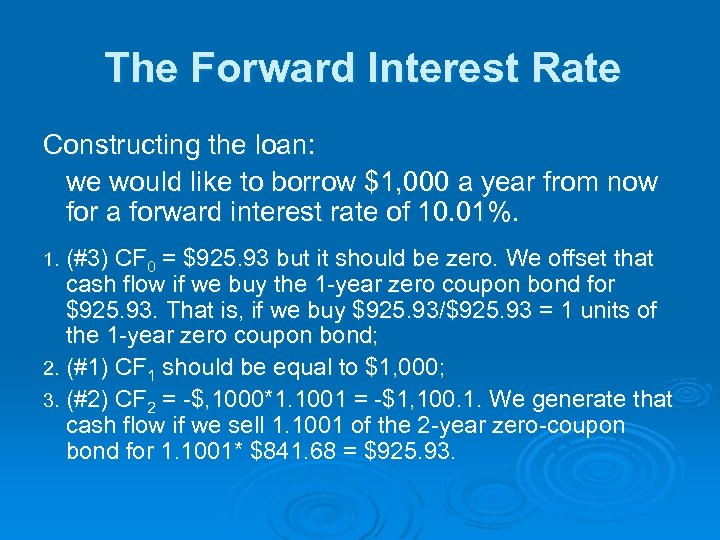The Forward Interest Rate Constructing the loan: we would like to borrow \$1, 000 a year from now for a forward interest rate of 10. 01%. 1. (#3) CF 0 = \$925. 93 but it should be zero. We offset that cash flow if we buy the 1 -year zero coupon bond for \$925. 93. That is, if we buy \$925. 93/\$925. 93 = 1 units of the 1 -year zero coupon bond; 2. (#1) CF 1 should be equal to \$1, 000; 3. (#2) CF 2 = -\$, 1000*1. 1001 = -\$1, 100. 1. We generate that cash flow if we sell 1. 1001 of the 2 -year zero-coupon bond for 1. 1001* \$841. 68 = \$925. 93.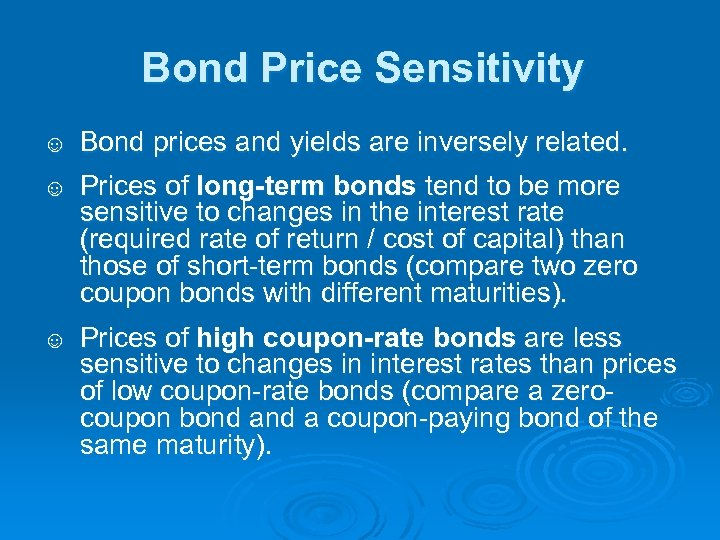Bond Price Sensitivity ☺ Bond prices and yields are inversely related. ☺ Prices of long-term bonds tend to be more sensitive to changes in the interest rate (required rate of return / cost of capital) than those of short-term bonds (compare two zero coupon bonds with different maturities). ☺ Prices of high coupon-rate bonds are less sensitive to changes in interest rates than prices of low coupon-rate bonds (compare a zerocoupon bond a coupon-paying bond of the same maturity).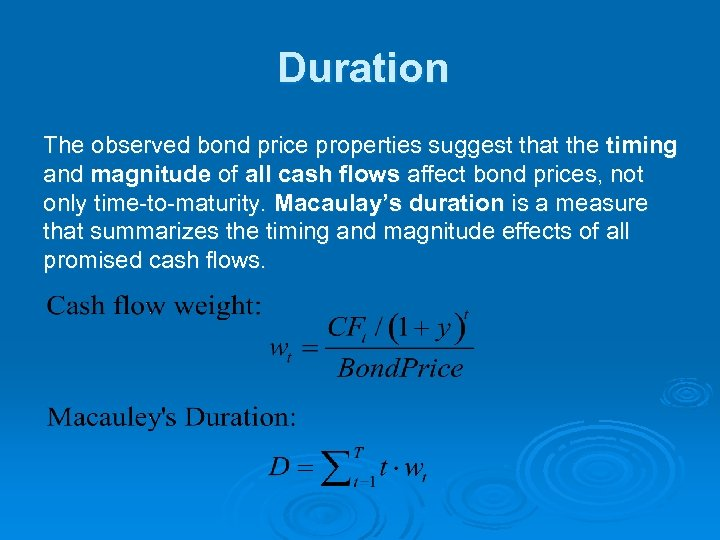Duration The observed bond price properties suggest that the timing and magnitude of all cash flows affect bond prices, not only time-to-maturity. Macaulay’s duration is a measure that summarizes the timing and magnitude effects of all promised cash flows.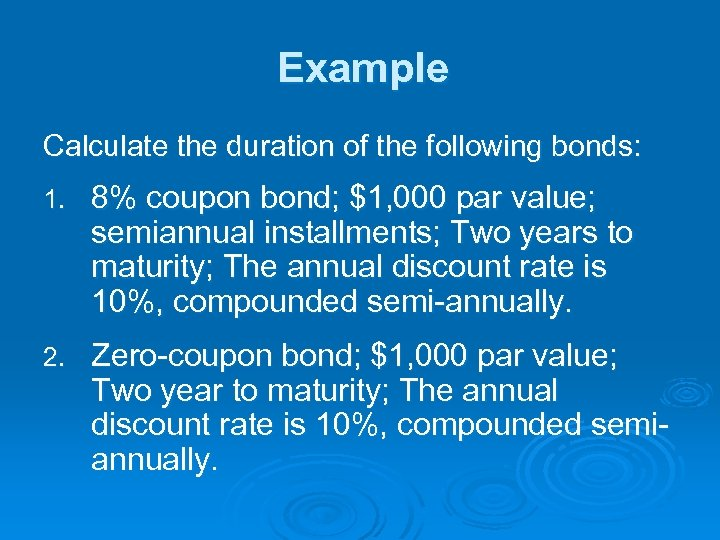Example Calculate the duration of the following bonds: 1. 8% coupon bond; \$1, 000 par value; semiannual installments; Two years to maturity; The annual discount rate is 10%, compounded semi-annually. 2. Zero-coupon bond; \$1, 000 par value; Two year to maturity; The annual discount rate is 10%, compounded semiannually.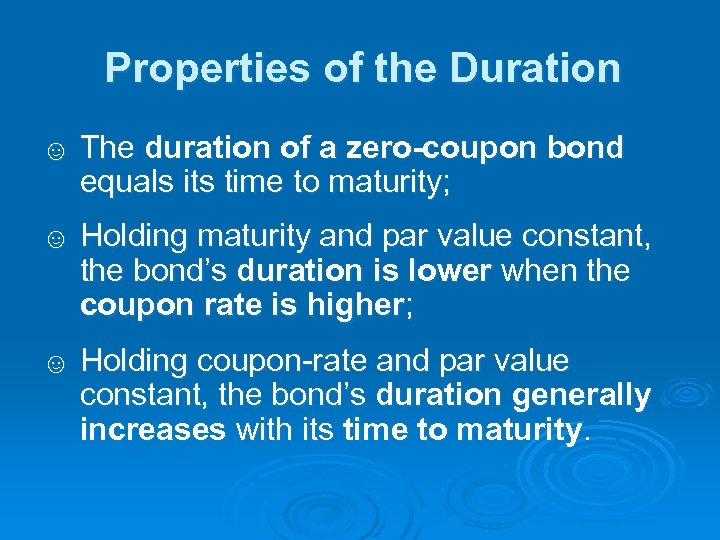Properties of the Duration ☺ The duration of a zero-coupon bond equals its time to maturity; ☺ Holding maturity and par value constant, the bond’s duration is lower when the coupon rate is higher; ☺ Holding coupon-rate and par value constant, the bond’s duration generally increases with its time to maturity.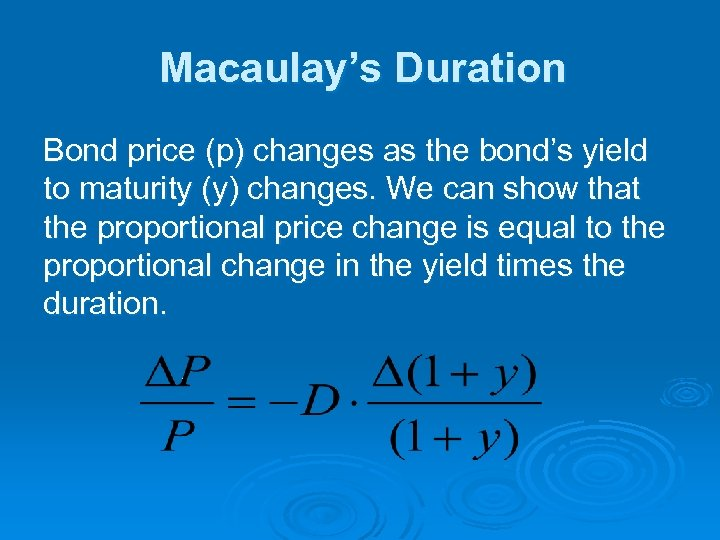Macaulay’s Duration Bond price (p) changes as the bond’s yield to maturity (y) changes. We can show that the proportional price change is equal to the proportional change in the yield times the duration.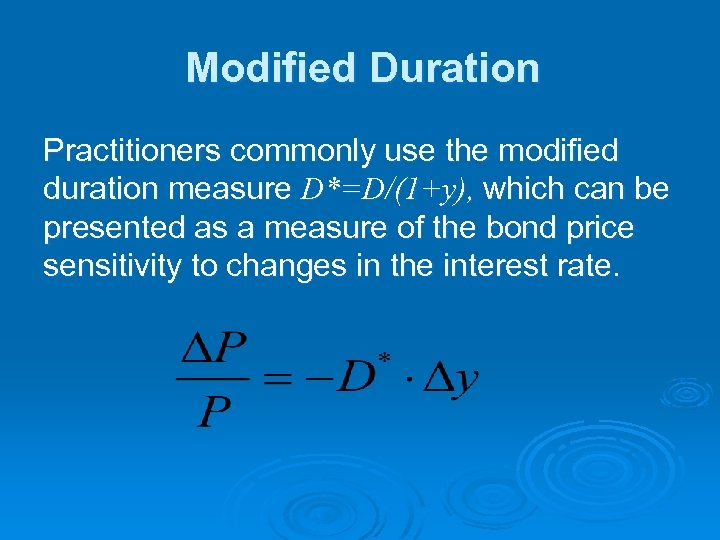Modified Duration Practitioners commonly use the modified duration measure D*=D/(1+y), which can be presented as a measure of the bond price sensitivity to changes in the interest rate.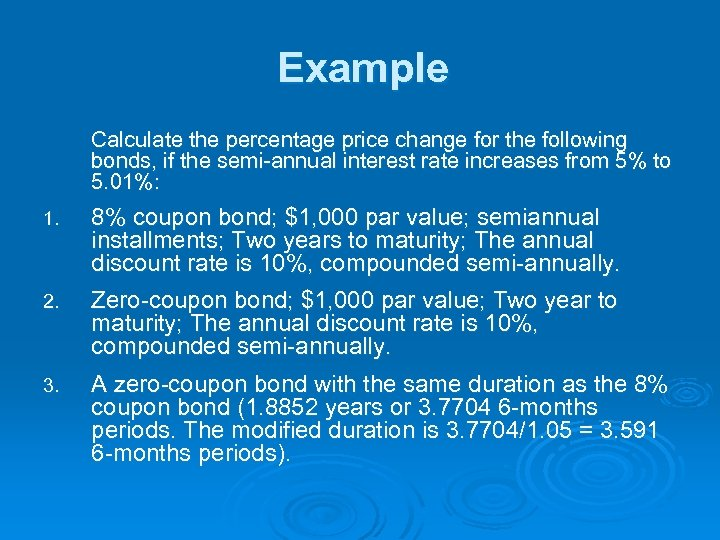Example Calculate the percentage price change for the following bonds, if the semi-annual interest rate increases from 5% to 5. 01%: 1. 8% coupon bond; \$1, 000 par value; semiannual installments; Two years to maturity; The annual discount rate is 10%, compounded semi-annually. 2. Zero-coupon bond; \$1, 000 par value; Two year to maturity; The annual discount rate is 10%, compounded semi-annually. 3. A zero-coupon bond with the same duration as the 8% coupon bond (1. 8852 years or 3. 7704 6 -months periods. The modified duration is 3. 7704/1. 05 = 3. 591 6 -months periods).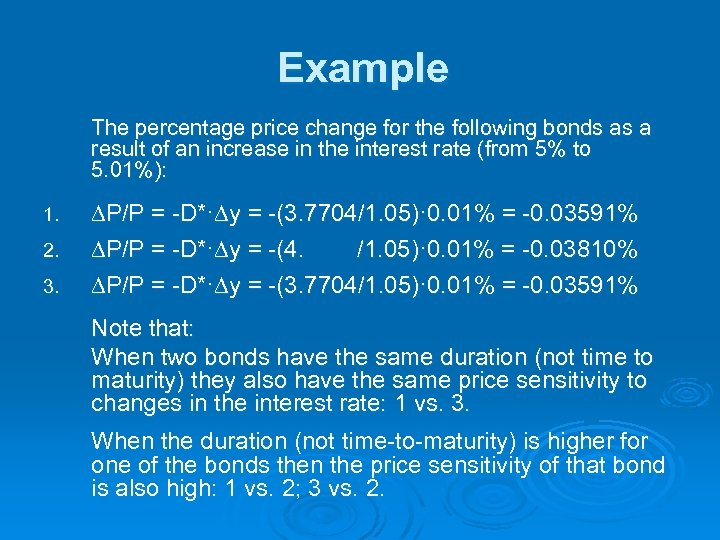Example The percentage price change for the following bonds as a result of an increase in the interest rate (from 5% to 5. 01%): 1. 2. 3. ∆P/P = -D*·∆y = -(3. 7704/1. 05)· 0. 01% = -0. 03591% ∆P/P = -D*·∆y = -(4. /1. 05)· 0. 01% = -0. 03810% ∆P/P = -D*·∆y = -(3. 7704/1. 05)· 0. 01% = -0. 03591% Note that: When two bonds have the same duration (not time to maturity) they also have the same price sensitivity to changes in the interest rate: 1 vs. 3. When the duration (not time-to-maturity) is higher for one of the bonds then the price sensitivity of that bond is also high: 1 vs. 2; 3 vs. 2.The Use of Duration ☺ It is a simple summary statistic of the effective average maturity of the bond (or portfolio of fixed income instruments); ☺ Duration can be presented as a measure of bond (portfolio) price sensitivity to changes in the interest rate (cost of capital); ☺ Duration is an essential tool in portfolio immunization: hedging interest rate risk.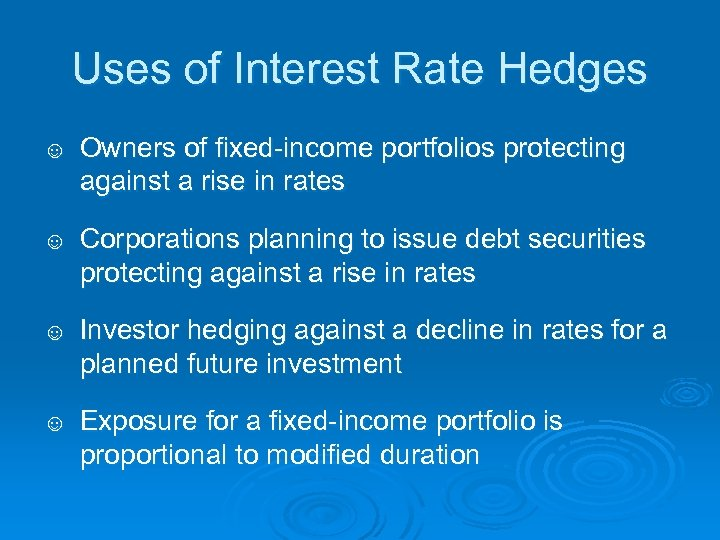Uses of Interest Rate Hedges ☺ Owners of fixed-income portfolios protecting against a rise in rates ☺ Corporations planning to issue debt securities protecting against a rise in rates ☺ Investor hedging against a decline in rates for a planned future investment ☺ Exposure for a fixed-income portfolio is proportional to modified duration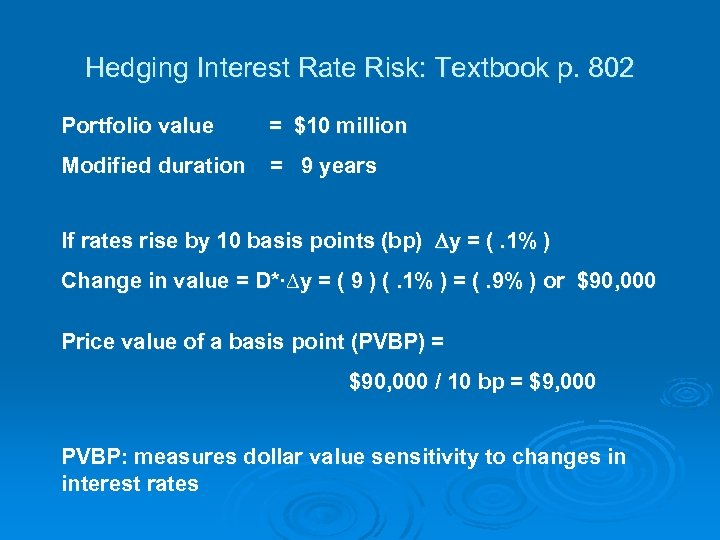Hedging Interest Rate Risk: Textbook p. 802 Portfolio value = \$10 million Modified duration = 9 years If rates rise by 10 basis points (bp) y = (. 1% ) Change in value = D*·∆y = ( 9 ) (. 1% ) = (. 9% ) or \$90, 000 Price value of a basis point (PVBP) = \$90, 000 / 10 bp = \$9, 000 PVBP: measures dollar value sensitivity to changes in interest rates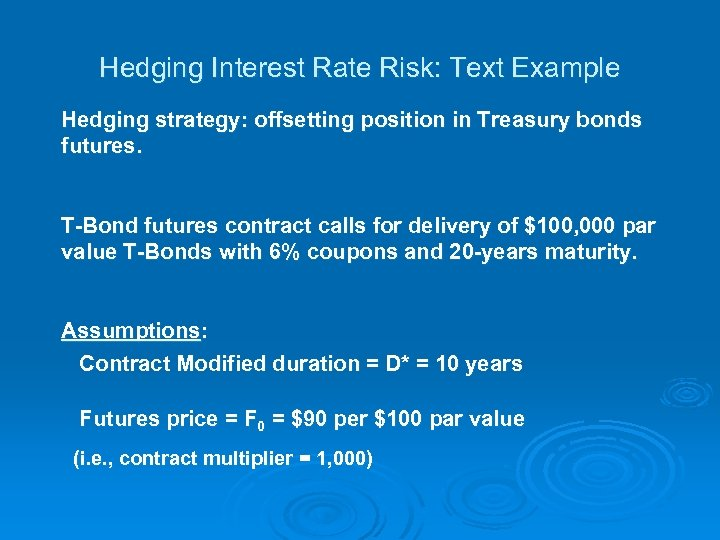Hedging Interest Rate Risk: Text Example Hedging strategy: offsetting position in Treasury bonds futures. T-Bond futures contract calls for delivery of \$100, 000 par value T-Bonds with 6% coupons and 20 -years maturity. Assumptions: Contract Modified duration = D* = 10 years Futures price = F 0 = \$90 per \$100 par value (i. e. , contract multiplier = 1, 000)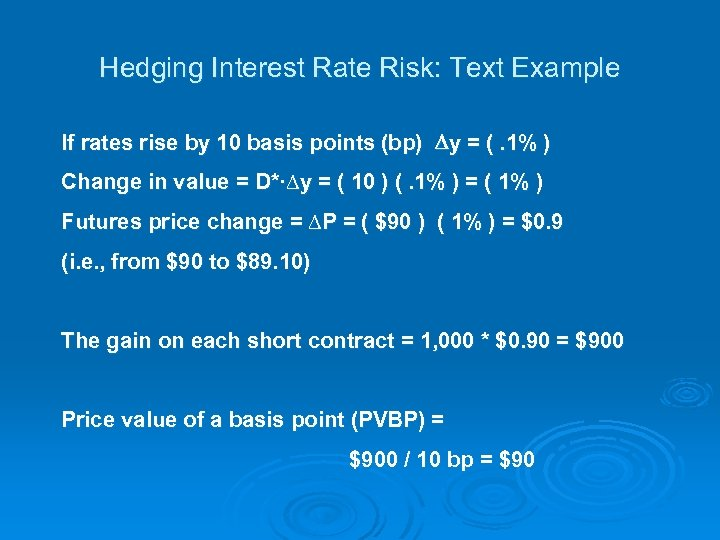Hedging Interest Rate Risk: Text Example If rates rise by 10 basis points (bp) y = (. 1% ) Change in value = D*·∆y = ( 10 ) (. 1% ) = ( 1% ) Futures price change = ∆P = ( \$90 ) ( 1% ) = \$0. 9 (i. e. , from \$90 to \$89. 10) The gain on each short contract = 1, 000 * \$0. 90 = \$900 Price value of a basis point (PVBP) = \$900 / 10 bp = \$90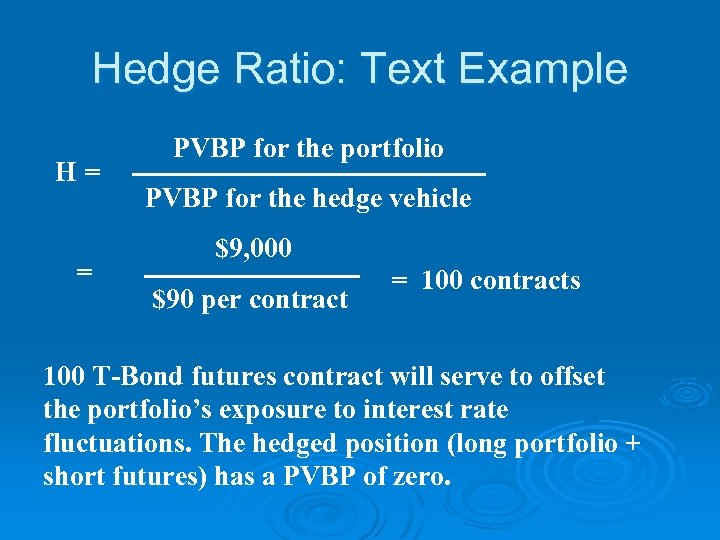Hedge Ratio: Text Example H= = PVBP for the portfolio PVBP for the hedge vehicle \$9, 000 \$90 per contract = 100 contracts 100 T-Bond futures contract will serve to offset the portfolio’s exposure to interest rate fluctuations. The hedged position (long portfolio + short futures) has a PVBP of zero.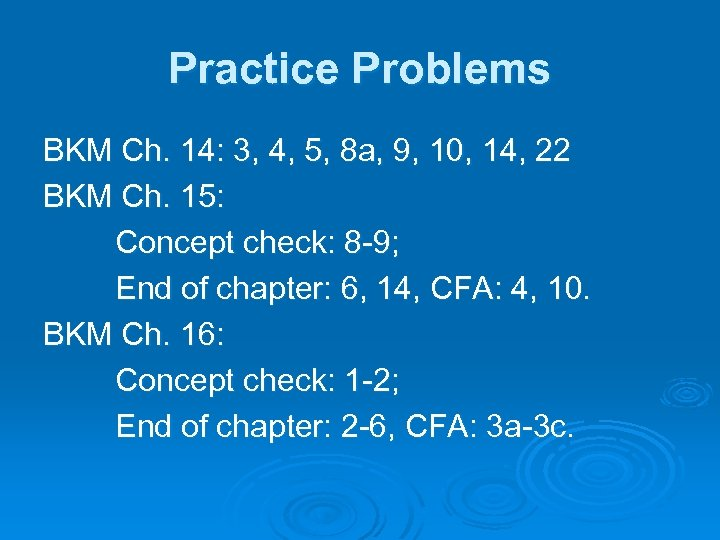Practice Problems BKM Ch. 14: 3, 4, 5, 8 a, 9, 10, 14, 22 BKM Ch. 15: Concept check: 8 -9; End of chapter: 6, 14, CFA: 4, 10. BKM Ch. 16: Concept check: 1 -2; End of chapter: 2 -6, CFA: 3 a-3 c.